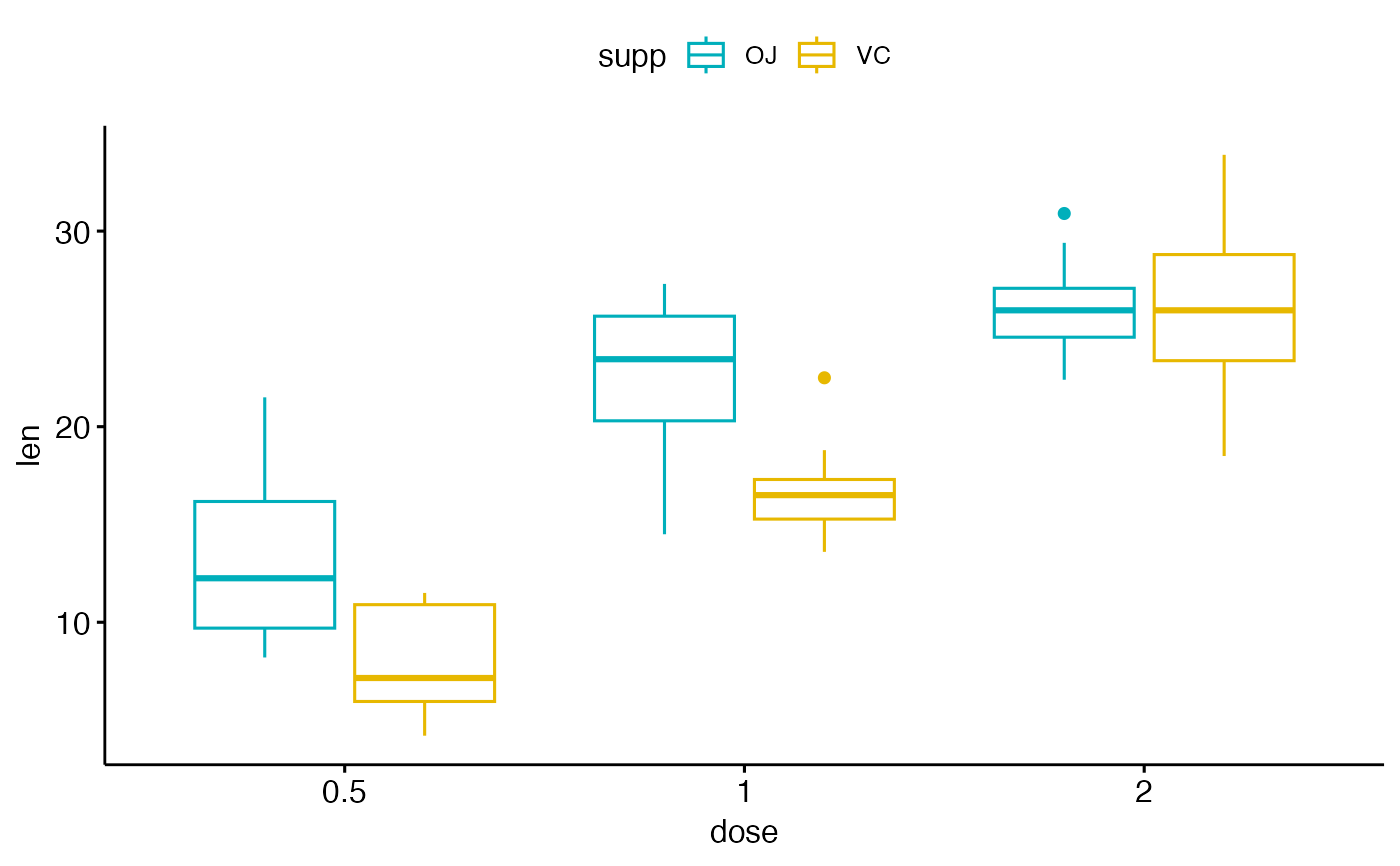Create a box plot with points. Box plots display a group of numerical data through their quartiles.

ggboxplot(
data,
x,
y,
combine = FALSE,
merge = FALSE,
color = "black",
fill = "white",
palette = NULL,
title = NULL,
xlab = NULL,
ylab = NULL,
bxp.errorbar = FALSE,
bxp.errorbar.width = 0.4,
facet.by = NULL,
panel.labs = NULL,
short.panel.labs = TRUE,
linetype = "solid",
size = NULL,
width = 0.7,
notch = FALSE,
outlier.shape = 19,
select = NULL,
remove = NULL,
order = NULL,
error.plot = "pointrange",
label = NULL,
font.label = list(size = 11, color = "black"),
label.select = NULL,
repel = FALSE,
label.rectangle = FALSE,
ggtheme = theme_pubr(),
...
)

## Arguments

data

a data frame

x

character string containing the name of x variable.

y

character vector containing one or more variables to plot

combine

logical value. Default is FALSE. Used only when y is a vector containing multiple variables to plot. If TRUE, create a multi-panel plot by combining the plot of y variables.

merge

logical or character value. Default is FALSE. Used only when y is a vector containing multiple variables to plot. If TRUE, merge multiple y variables in the same plotting area. Allowed values include also "asis" (TRUE) and "flip". If merge = "flip", then y variables are used as x tick labels and the x variable is used as grouping variable.

color

outline color.

fill

fill color.

palette

the color palette to be used for coloring or filling by groups. Allowed values include "grey" for grey color palettes; brewer palettes e.g. "RdBu", "Blues", ...; or custom color palette e.g. c("blue", "red"); and scientific journal palettes from ggsci R package, e.g.: "npg", "aaas", "lancet", "jco", "ucscgb", "uchicago", "simpsons" and "rickandmorty".

title

plot main title.

xlab

character vector specifying x axis labels. Use xlab = FALSE to hide xlab.

ylab

character vector specifying y axis labels. Use ylab = FALSE to hide ylab.

bxp.errorbar

logical value. If TRUE, shows error bars of box plots.

bxp.errorbar.width

numeric value specifying the width of box plot error bars. Default is 0.4.

facet.by

character vector, of length 1 or 2, specifying grouping variables for faceting the plot into multiple panels. Should be in the data.

panel.labs

a list of one or two character vectors to modify facet panel labels. For example, panel.labs = list(sex = c("Male", "Female")) specifies the labels for the "sex" variable. For two grouping variables, you can use for example panel.labs = list(sex = c("Male", "Female"), rx = c("Obs", "Lev", "Lev2") ).

short.panel.labs

logical value. Default is TRUE. If TRUE, create short labels for panels by omitting variable names; in other words panels will be labelled only by variable grouping levels.

linetype

line types.

size

Numeric value (e.g.: size = 1). change the size of points and outlines.

width

numeric value between 0 and 1 specifying box width.

notch

If FALSE (default) make a standard box plot. If TRUE, make a notched box plot. Notches are used to compare groups; if the notches of two boxes do not overlap, this suggests that the medians are significantly different.

outlier.shape

point shape of outlier. Default is 19. To hide outlier, specify outlier.shape = NA. When jitter is added, then outliers will be automatically hidden.

select

character vector specifying which items to display.

remove

character vector specifying which items to remove from the plot.

order

character vector specifying the order of items.

character vector for adding another plot element (e.g.: dot plot or error bars). Allowed values are one or the combination of: "none", "dotplot", "jitter", "boxplot", "point", "mean", "mean_se", "mean_sd", "mean_ci", "mean_range", "median", "median_iqr", "median_hilow", "median_q1q3", "median_mad", "median_range"; see ?desc_statby for more details.

parameters (color, shape, size, fill, linetype) for the argument 'add'; e.g.: add.params = list(color = "red").

error.plot

plot type used to visualize error. Allowed values are one of c("pointrange", "linerange", "crossbar", "errorbar", "upper_errorbar", "lower_errorbar", "upper_pointrange", "lower_pointrange", "upper_linerange", "lower_linerange"). Default value is "pointrange" or "errorbar". Used only when add != "none" and add contains one "mean_*" or "med_*" where "*" = sd, se, ....

label

the name of the column containing point labels. Can be also a character vector with length = nrow(data).

font.label

a list which can contain the combination of the following elements: the size (e.g.: 14), the style (e.g.: "plain", "bold", "italic", "bold.italic") and the color (e.g.: "red") of labels. For example font.label = list(size = 14, face = "bold", color ="red"). To specify only the size and the style, use font.label = list(size = 14, face = "plain").

label.select

can be of two formats:

• a character vector specifying some labels to show.

• a list containing one or the combination of the following components:

• top.up and top.down: to display the labels of the top up/down points. For example, label.select = list(top.up = 10, top.down = 4).

• criteria: to filter, for example, by x and y variabes values, use this: label.select = list(criteria = "y > 2 & y < 5 & x %in% c('A', 'B')").

repel

a logical value, whether to use ggrepel to avoid overplotting text labels or not.

label.rectangle

logical value. If TRUE, add rectangle underneath the text, making it easier to read.

ggtheme

function, ggplot2 theme name. Default value is theme_pubr(). Allowed values include ggplot2 official themes: theme_gray(), theme_bw(), theme_minimal(), theme_classic(), theme_void(), ....

...

other arguments to be passed to geom_boxplot, ggpar and facet.

## Details

The plot can be easily customized using the function ggpar(). Read ?ggpar for changing:

• main title and axis labels: main, xlab, ylab

• axis limits: xlim, ylim (e.g.: ylim = c(0, 30))

• axis scales: xscale, yscale (e.g.: yscale = "log2")

• color palettes: palette = "Dark2" or palette = c("gray", "blue", "red")

• legend title, labels and position: legend = "right"

• plot orientation : orientation = c("vertical", "horizontal", "reverse")

## Suggestions for the argument "add"

Suggested values are one of c("dotplot", "jitter").

ggpar, ggviolin, ggdotplot and ggstripchart.

## Examples

# Load data
data("ToothGrowth")
df <- ToothGrowth

# Basic plot
# +++++++++++++++++++++++++++
# width: change box plots width
ggboxplot(df, x = "dose", y = "len", width = 0.8)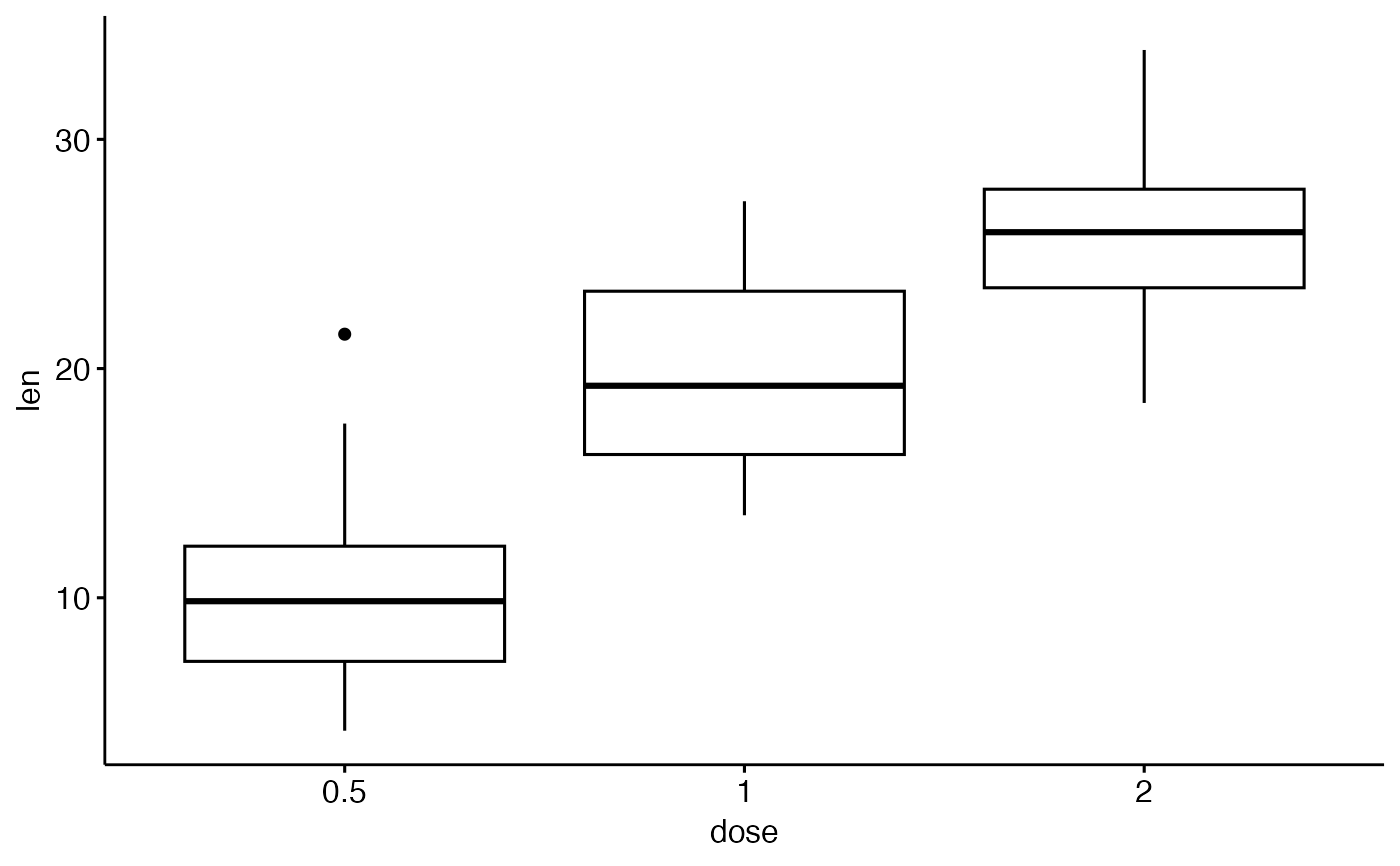# Change orientation: horizontal
ggboxplot(df, "dose", "len", orientation = "horizontal")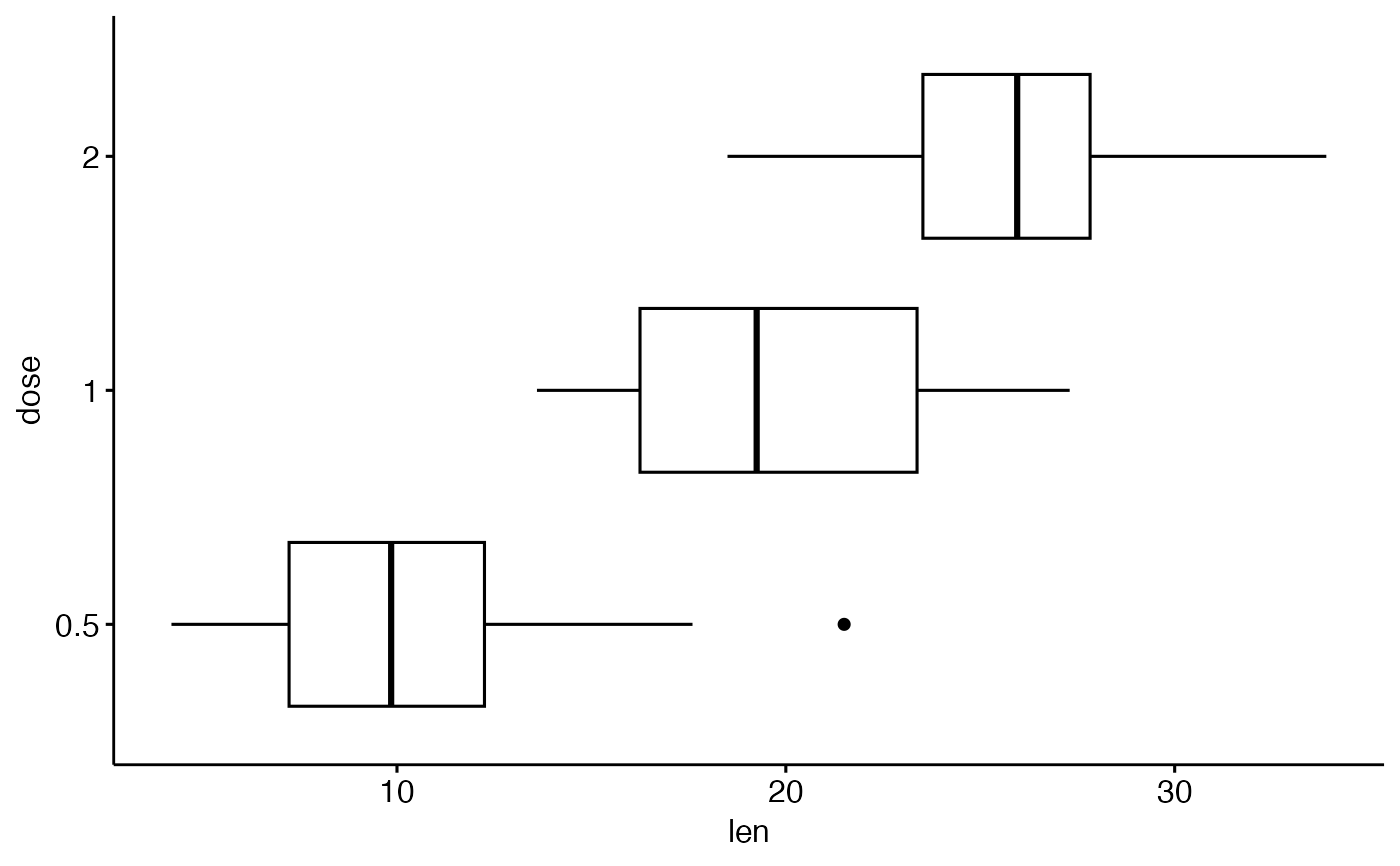# Notched box plot
ggboxplot(df, x = "dose", y = "len",
notch = TRUE)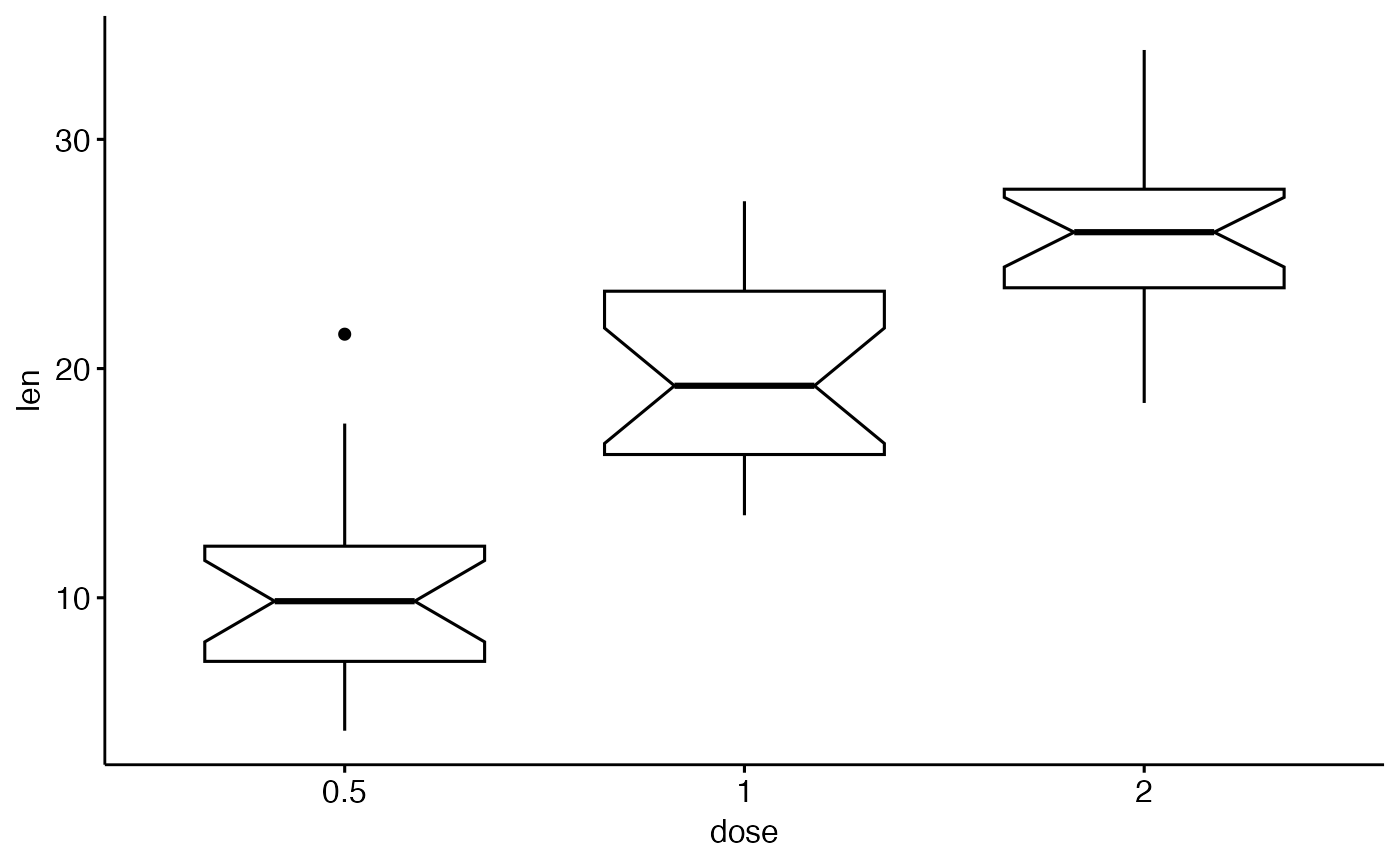# ++++++++++++++++++++++++++
ggboxplot(df, x = "dose", y = "len",
#> Bin width defaults to 1/30 of the range of the data. Pick better value with
#> binwidth.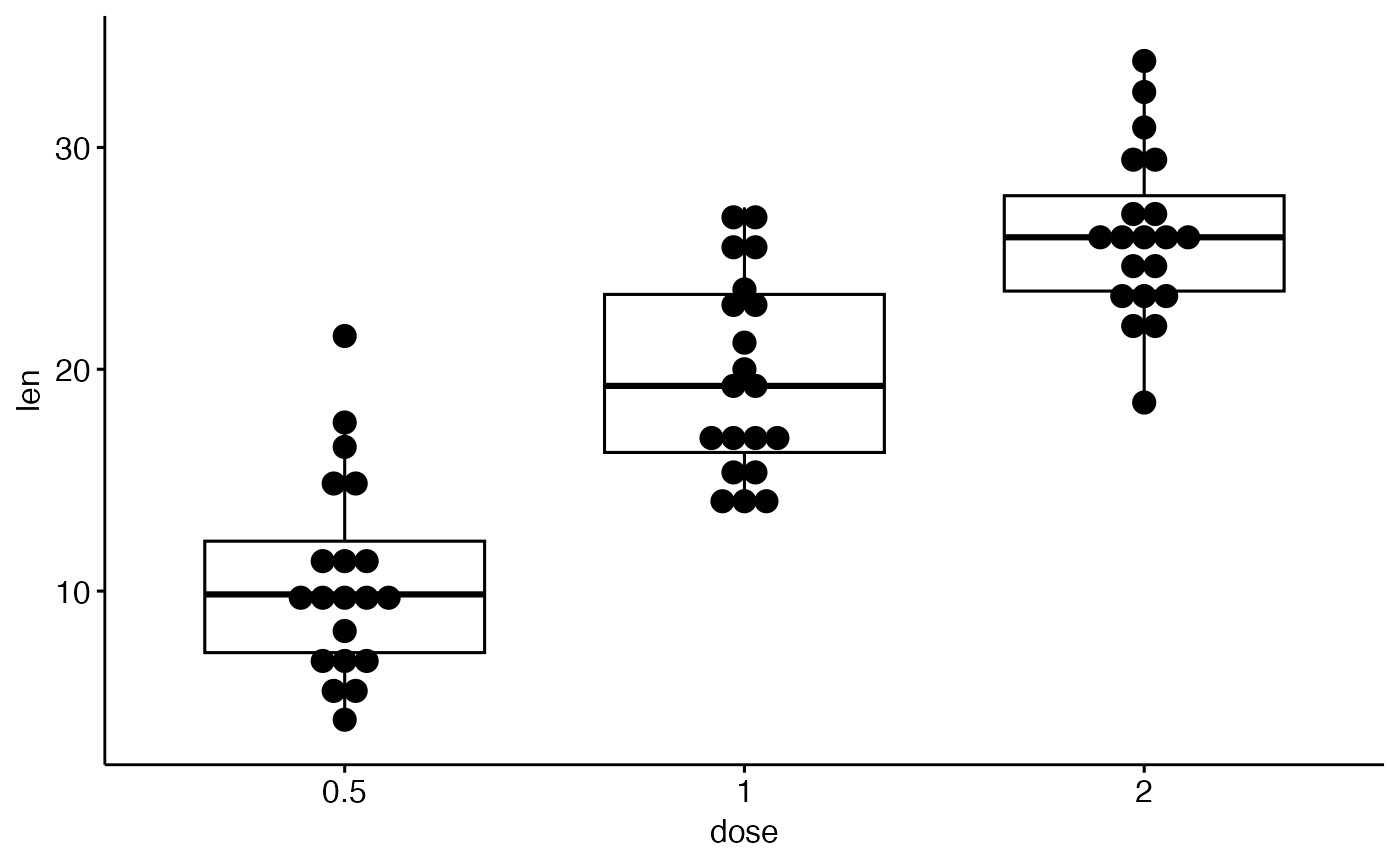# Add jitter points and change the shape by groups
ggboxplot(df, x = "dose", y = "len",
add = "jitter", shape = "dose")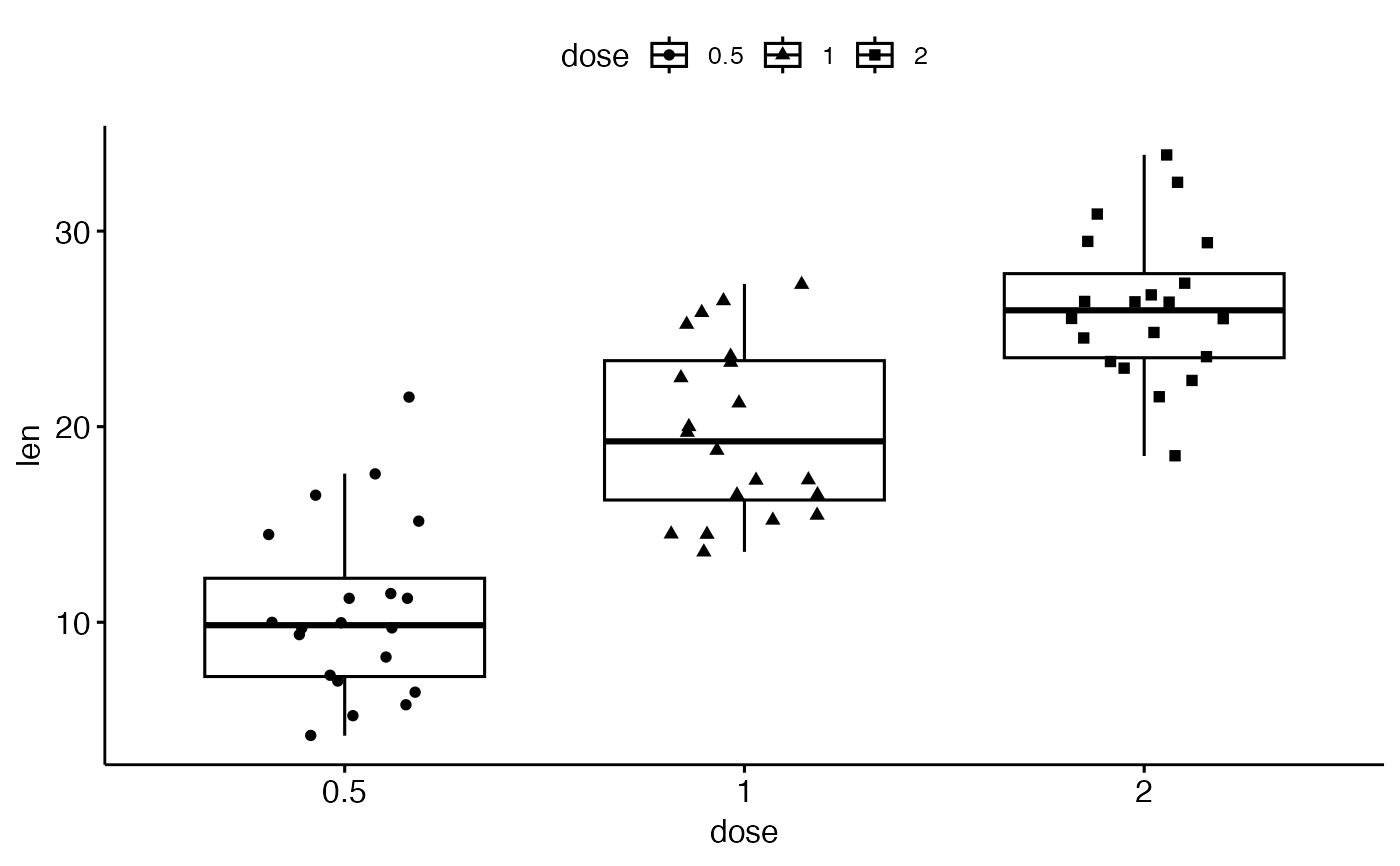# Select and order items
# ++++++++++++++++++++++++++++++

# Select which items to display: "0.5" and "2"
ggboxplot(df, "dose", "len",
select = c("0.5", "2"))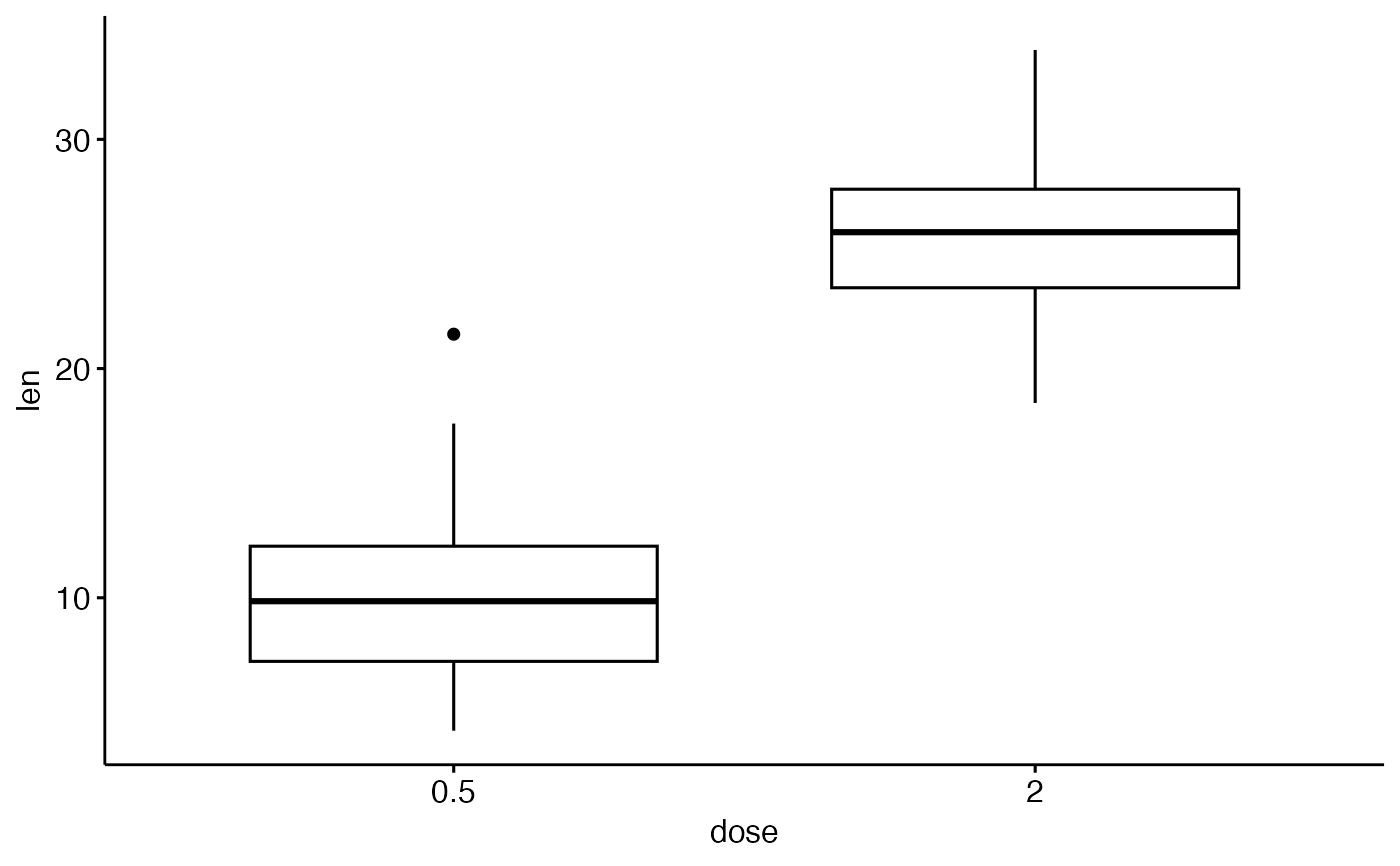# Change the default order of items
ggboxplot(df, "dose", "len",
order = c("2", "1", "0.5"))# Change colors
# +++++++++++++++++++++++++++
# Change outline and fill colors
ggboxplot(df, "dose", "len",
color = "black", fill = "gray")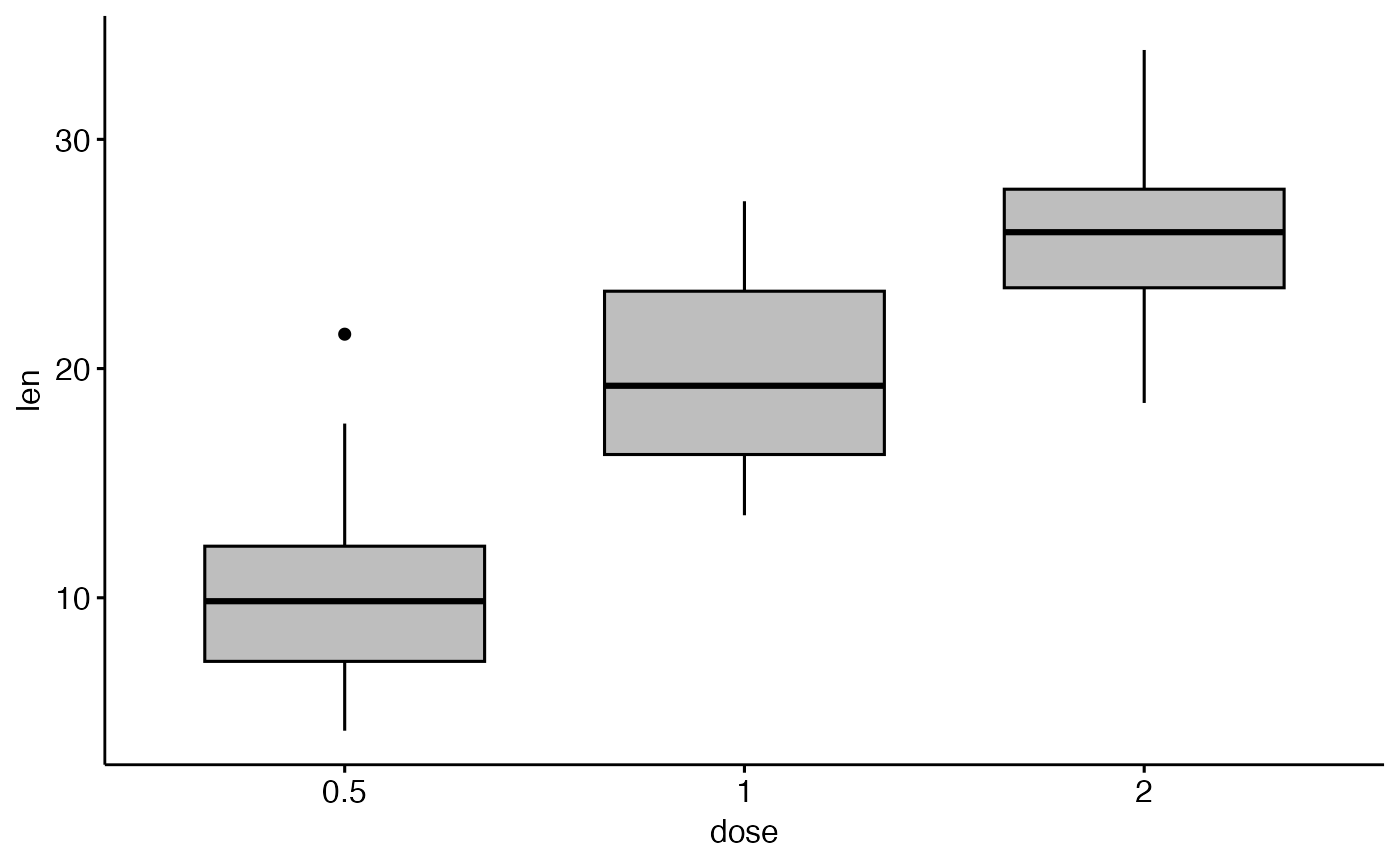# Change outline colors by groups: dose
# Use custom color palette
# Add jitter points and change the shape by groups
ggboxplot(df, "dose", "len",
color = "dose", palette =c("#00AFBB", "#E7B800", "#FC4E07"),
add = "jitter", shape = "dose")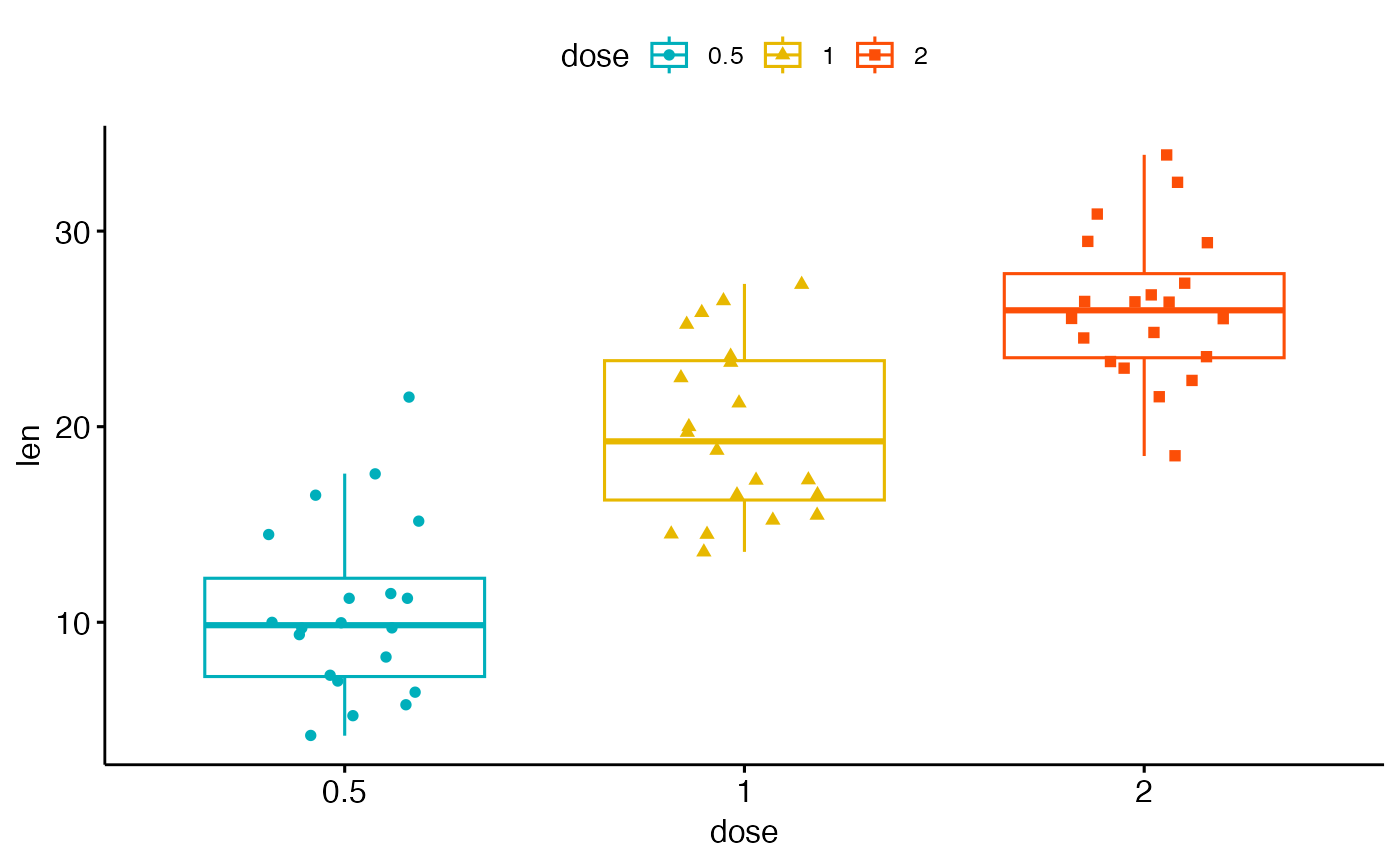# Change fill color by groups: dose
ggboxplot(df, "dose", "len",
fill = "dose", palette = c("#00AFBB", "#E7B800", "#FC4E07"))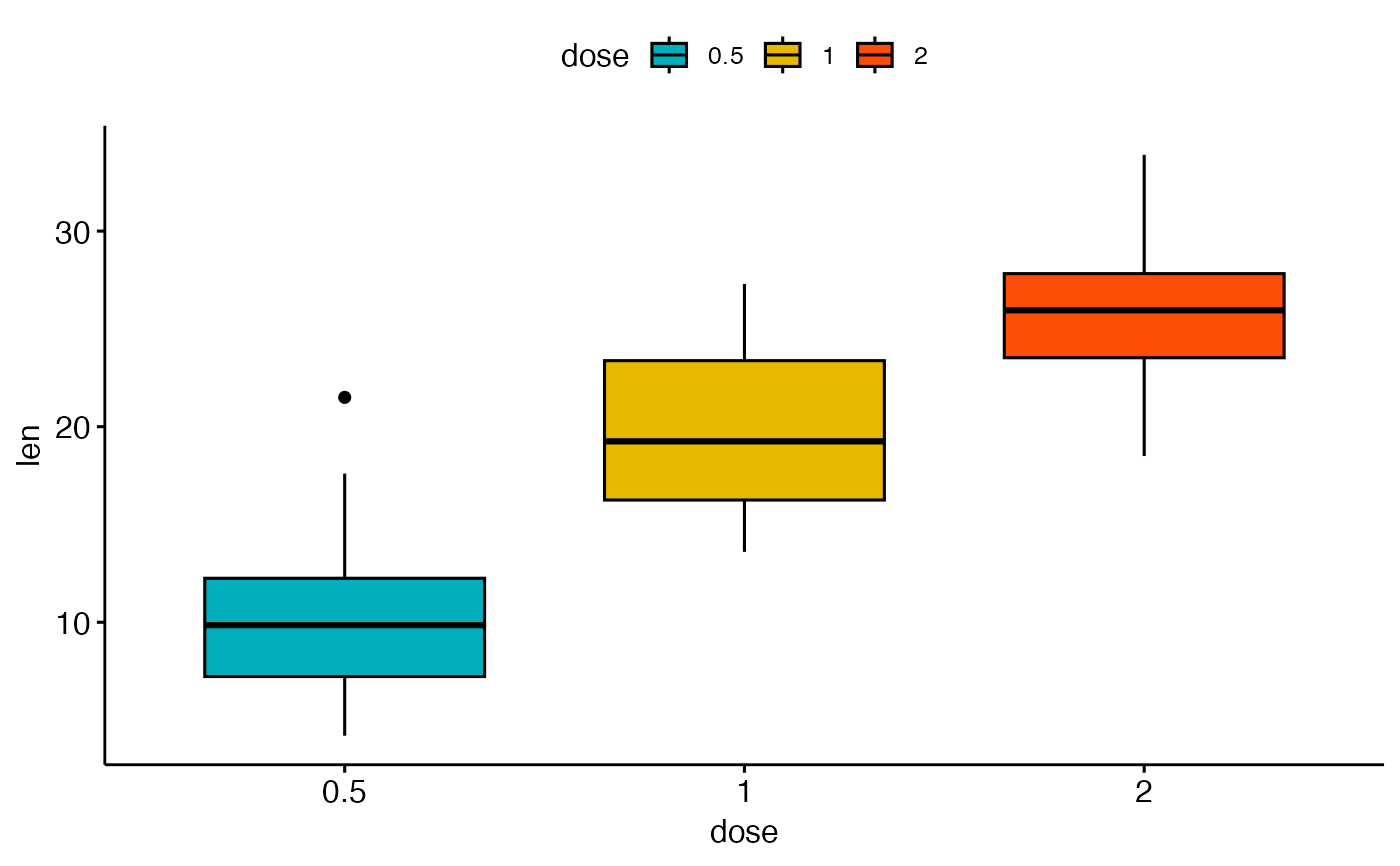# Box plot with multiple groups
# +++++++++++++++++++++
# fill or color box plot by a second group : "supp"
ggboxplot(df, "dose", "len", color = "supp",
palette = c("#00AFBB", "#E7B800"))